# 7: Making Decisions With JavaScript

### Objectives

In this Tutorial you will learn how to:

• Create decision-making structures in JavaScript with if, else if and else code blocks.

In this Tutorial, you will meet the following terms:

• Comparison operator
• Logical operator

### Exercise Files

In this Tutorial, you will work with the following HTML files:

Unzip the five files it contains into a sub-folder named /7.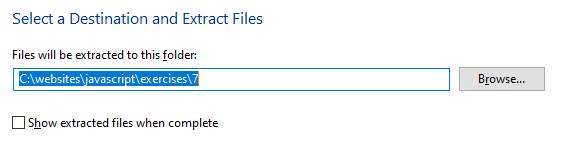## Decision-making with if

Can computers make decisions? Or can they do only what they are told?

The answer to both questions is: 'Yes'.

A computer can make decisions if that's what the software tells it to do. In JavaScript, as in other programming and scripting languages, the if keyword is at the basis of most decision-making. The code structure is as follows:

```if (condition is true) {
perform some instruction(s);
}```

Note the following points about the if code structure:

• The statement begins with the keyword if – not IF or If or iF.
• The condition tested by the if statement is enclosed within parenthesis ().
• The statement that is performed if the condition is true is written inside a pair of opening and closing curly braces { }.

What is meant by the word 'condition' In this context, a condition is a combination of :

• A literal value or variable, or two variables, and
• An operator such as 'equal to' or 'greater than' that defines the relationship between the two.

### The if statement in action

Consider the following example that tests the value in the variable named 'temperature'. If the value in the variable exceeds a certain amount, the condition is satisfied and the script shows an alert box. The right angle bracket (>) is the JavaScript greater than operator.

```let temperature = 21;
if (temperature > 15) {
window.alert("Temperature is greater than 15 degrees");
}```

Here are some other possible conditions that a script could test. The first example tests if the temperature variable is less than the value of 15.

```if (temperature < 15)
```

The condition below tests if the temperature variable is equal to the value of 15.

```if (temperature == 15)
```

The next example tests if the temperature variable is greater than or equal to the value of 15.

```if (temperature >= 15)
```

This example below tests if the temperature variable is less than or equal to the value of 15.

```if (temperature <= 15)
```

Finally, the example below tests if the temperature variable is not equal to the value of 15.

```if ( temperature != 15)
```

In Exercise 7.1 you will create a simple script that accepts a user-entered number in a window.prompt() box, assigns it to a variable, tests the value of a variable, and writes a text message to a window.alert() box if the variable satisfies a particular condition.

### Exercise 7.1: Using a simple if statement

Open the following file in VS Code and web browser:

exercise-7-1.html

After the opening <script> tag enter the following code.

```let userNumber;
userNumber = window.prompt("Please enter a number between 1 and 100.");
```

Beneath the above two lines enter the following if statement.

```if (userNumber < 50 ) {
}```

Enter a number less than 50 (say, 25) in the box when prompted, click OK, and note the result shown in the dialog box.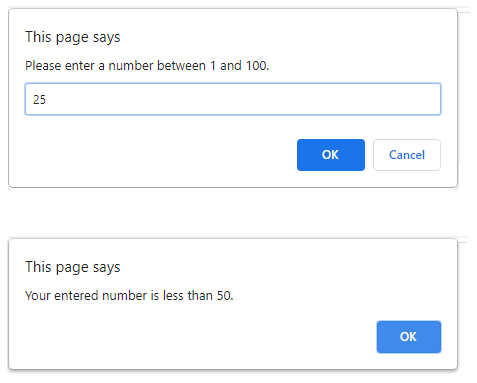You can close the exercise-7-1.html file when finished.

## Comparison operators

When testing a condition in JavaScript a comparison operator is used to make some kind of comparison between two values. The following table describes the main comparison operators.

 Operator Symbol Description == Tests if the two values are the same. != Tests if the two values are not the same. > Tests if the first value is greater than the second. >= Tests if the first value is greater than or equal to the second. < Tests if the first value is less than the second. <= Tests if the first value is less than or equal to the second.

Comparison Operator

An operator that can compare two values. Such operators are typically used to control script flow as part of if statements.

### Handling more than one condition

A limitation of the script you created in Exercise 7.1 is that it provides feedback to the web page visitor only when the entered a number that is less than the tested value – that is, when the number is less than 50.

It is good practice to provide user feedback in all situations.

One way to do this is to combine an if command with the else if command in a single JavaScript code block. You can then evaluate more than two possible outcomes.

Here is a basic example.

```if (first condition true) {
perform first instruction;
}

else if (second condition true) {
perform second other instruction;
}```

In Exercise 7.2 you will improve the script in the first exercise by adding an else if branch.

### Exercise 7.2: Using the if and else if structure

exercise-7-2.html

It is a script similar to the one you created in the previous exercise and contains a single if statement.

However, it now contains two instructions to display a window.alert() box, each one with a different message.

```if (userNumber < 50 ) {
}

else if (userNumber > 50 ) {
}```

What happens if a visitor to the web page enters the number '50'?

To handle this situation, add a second else if statement to cope with this situation.

Your complete code block should now look as shown below.

```if (userNumber < 50 ) {
}

else if (userNumber > 50 ) {
}

else if (userNumber == 50 ) {
}```

Test your script by entering various numbers between 1 and 100 in the box when prompted, click OK, and note the results displayed in the window.alert() box.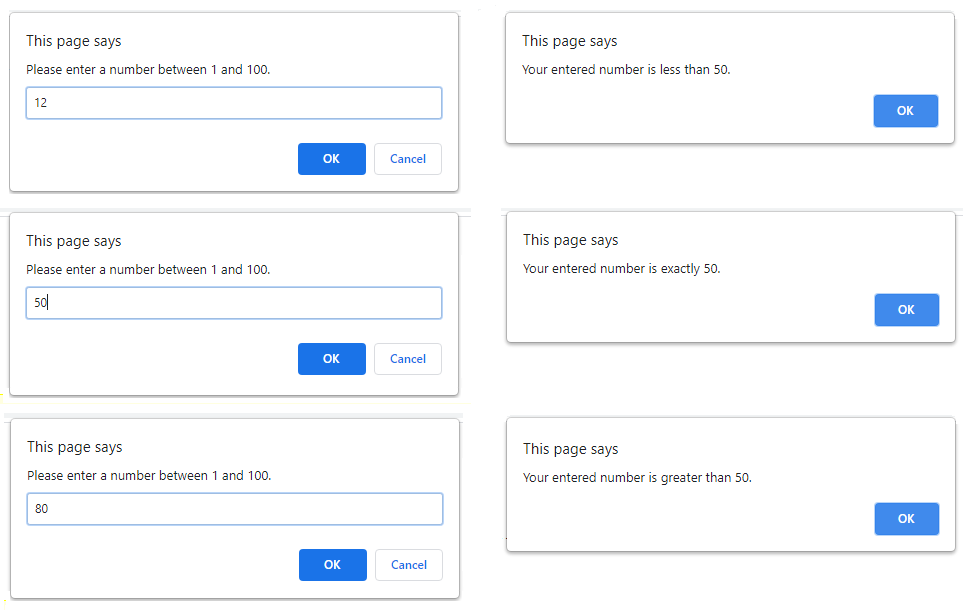You can close the exercise-7.2.html file when finished.

## The all-purpose else command

If you have ever organised physical items in a room or workplace you probably ended up with a drawer or shelf called 'miscellaneous' or 'other stuff'.

Similarly, there are many scripting situations where no amount of if and else if statements is enough to cope with every possible value that may be entered by a web page visitor.

The remedy is to use the else command. This is JavaScript's way of handling values that fall in the category of 'other' or 'miscellaneous'.

The general form of the if, if else and else decision-making structure is as follows.

```if (condition is true) {
perform first instruction;
}

else if (condition is true) {
perform second instruction;
}

else {
perform some other instruction;
}```

### Exercise 7.3: Using the else command

exercise-7-3.html

The script works as follows:

• Web page visitors are prompted to type their country name in a text box.
• Your code assigns the entered country name to a variable named 'userCountry'.
```  let userCountry = document.getElementById("countryName").value;
```
• The code declares a variable named 'userGreeting' and stores the appropriate greeting text with a flag emoji character in it.
```   let userGreeting;

if userCountry == "France") {
userGreeting = "🇫🇷  Bonjour";
}

else if userCountry == "Germany") {
userGreeting = "🇩🇪  Guten tag";
}

else if userCountry == "Italy") {
userGreeting = "🇮🇹  Ciao";
}```
• At the bottom of the web page is an event listener that connects the user action of clicking the button (id of btnUserCountry) with calling the function (name of userCountryGreeting)
• Your web page displays the appropriate greeting in the div tag with an id of resultBox response.
```  document.getElementById("resultBox").innerHTML = userGreeting;
```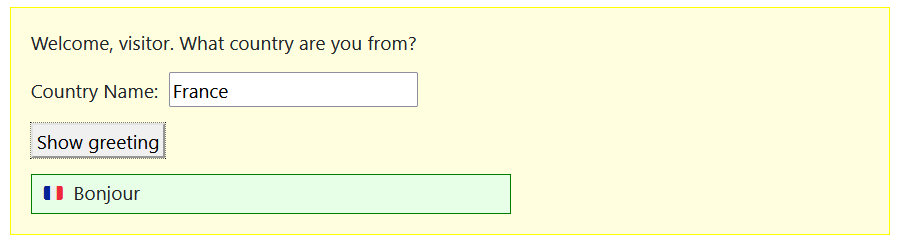But what if someone enters the name of a country that is not one of the above – that is, not Italy, Germany or France?

The script has no way of handling that situation.

In this example, insert the following code after the final else if statement.

```else {
userGreeting = "🌎  Wherever you are from, welcome to my website";
}```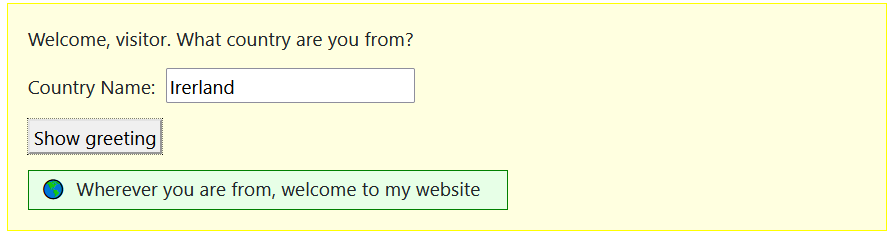You can close the exercise-7.3.html file when finished.

## Linking conditions with logical operators

There are times when one condition is not enough. You can join multiple conditions together to form a single statement using the so-called logical operators.

The following code tests both temperature and humidity with the AND (&&) operator.

```let temperature = 21;
let humidity = 61;
if ( (temperature > 20) && (humidity > 60) ) {
console.log("Temperature and humidity conditions are met");
}```

The following code uses the OR (||) operator and is satisfied if either one condition or both conditions are met.

```let temperature = 19;
let humidity = 61;
if ( (temperature > 20) || (humidity > 60) ) {
console.log("Yes. One or both are met" );
}```

The following table describes the two logical operators.

 Operator Symbol Description && The AND operator. Joins multiple conditions in such a way that the output is true only if all the individual conditions are true. || The OR operator. Joins multiple conditions in such a way that the output is true if any one (or more or all) of the individual conditions is true.

Logical Operator

An operator that can compare two values. Such operators typically used to control script flow as part of if statements.

In Exercise 7.4 you will use the AND logical operator to test the username and password entered by a web page visitor. Please note that is script is only for demonstration. It is not secure because the correct values are visible to anyone who reads the script.

### Exercise 7.4: Building a simple user access web page

exercise-7-4.html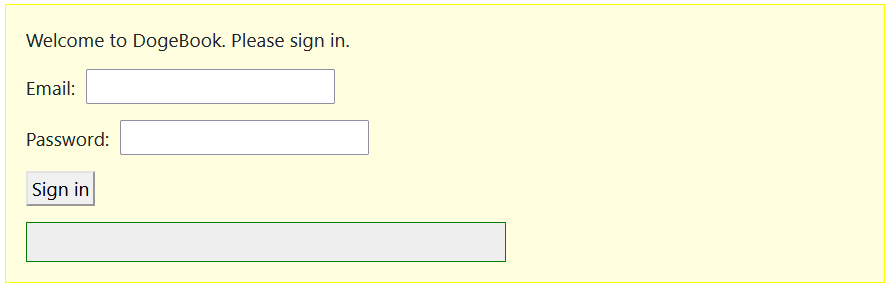In the file is a function named checkUser() that contains only comments.

You can also see two required input fields ( inputEmail and inputPwd ), a div element (id of resultBox ) for displaying an output message, a button (id of btnSignin) and an event listener.

Inside the function, insert the following variable declarations and comments.

```// Declare variables
let userEmail = "patrick99@dogemail.com"; // from database
let userPwd = "abc123"; // from database
let message;
```

Next, insert the following statements that assign the user-entered field values to JavaScript variables.

```// Assign user inputs to variables
let inputEmail = document.getElementById("inputEmail").value;
let inputPwd   = document.getElementById("inputPwd").value;
```

Insert the following if…else structure with the && statement.

```// Test if both inputs correct
if ( (inputEmail == userEmail) && (inputPwd == userPwd) ) {
message = "✅  Details correct. Please continue."
}

// Oops. One or both inputs incorrect
else {
message = "❌  Details incorrect. Please try again."
}
document.getElementById("resultBox").innerHTML = message;```

Test your script by entering various combinations of correct and incorrect usernames and passwords. You can close the file when finished.

In Exercise 7.5 you will use the if else structure in combination with an AND && operator to improve the script you created in Exercise 7.2.

### Exercise 7.5: Improving your user input test web page

Open the following file in VS Code and web browser:

exercise-7-5.html

It is a script similar to the one you created in Exercise 7.2. Visitors to this web page are asked to enter a number in the range 1 to 100, and the script can display one of three messages in response.

But what if someone enters 110, 456768 or -24.4?

As a first step. insert a new if statement before the existing one as shown below.

```if ( (userNumber < 0 ) || (userNumber > 100 ) ) {
}```

Next, change what was the first if statement to an else ifstatement, as shown below.

```else if (userNumber < 50 ) {
}
```

Test your new code by enter numbers less than 1 and greater than 100.

Try entering a number that contains a comma separator (such as 12,500) and note that the script does not respond. That is because such a number is treated as a string.

You can close the exercise-7-5.html file when finished.

1. Sign in to Github and click the name of the repository (‘repo’) that holds your web pages.
2. On the next GitHub screen displayed, click the Add file button near the right of the screen and then choose Upload files from the dropdown list.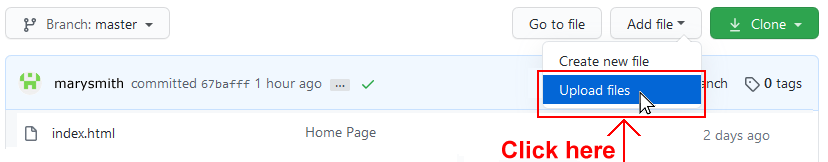3. In File Explorer (Windows 10) or Finder (Apple Mac), select your entire 📁 /javascript sub-folder, and drag-and-drop it to your repository on GitHub.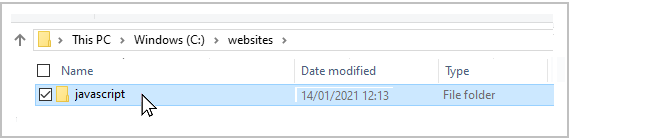4. Scroll down to the bottom of the GitHub screen, and accept or edit the short message (Add files via upload) in the Commit changes box.   Finally, click the green Commit changes button to upload your entire 📁 /javascript sub-folder and all the exercise files it contains.

It may take a few minutes for your uploaded files to appear on GitHub.

## Lesson Summary

In JavaScript as in other programming and scripting languages the if command is at the basis of most decision-making. The syntax is as follows:

```if (condition is true) {
perform instruction;
}
```

The condition can include a literal value, a variable, or two with comparison operator such as ‘equal to’ or ‘greater than’ that defines the relationship between the two. For situations where multiple if statements cannot cope with all possible variable values, JavaScript provides an else command. Its syntax is as follows.

```if (condition is true) {
perform instruction;
}
else {
perform other instruction;
}```

You can join multiple conditions together to form a single statement using the so-called logical operators, AND (&&) and OR (||). An alternative to using one or more if commands, perhaps ending with a ‘catch all’ else command, is provided by the else if command. The general syntax is as follows.

```if (first condition true) {
perform first instruction;
}
else if (second condition true) {
perform second other instruction;
}
else if (third condition true) {
perform third instruction;
}
else {
perform some other instruction;
}
```